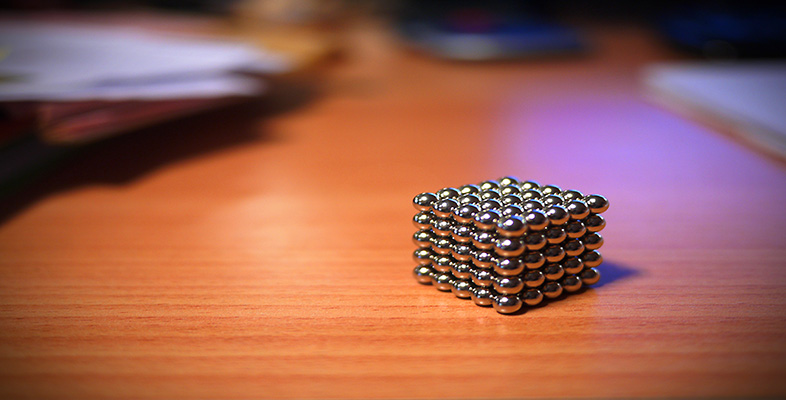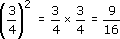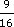Squares, roots and powers

This free course is available to start right now. Review the full course description and key learning outcomes and create an account and enrol if you want a free statement of participation.

Free course

1.2 Squaring fractions and negative numbers

You have now seen how to find squares of whole numbers and decimals. What about fractions? The rule is as before: to square a fraction, just multiply it by itself.

For example:In Example 1, you could have usedinstead of 0.75. Check on your calculator that 0.752 =. Both answers should be 0.5625.

There may be contexts where you need to square negative numbers. Take care when doing so. Use the brackets if in doubt. Calculating by hand gives, for example:

(9)2 = 9 × 9 = 81

However, keying in 9 and then squaring will give 81 on many scientific and graphics calculators. Why? They calculate exactly what you have asked for, i.e. 92. Since 92 is 81, 92 is 81. The number 9 is squared first, to give 81, and then made negative, to give 81. Brackets are needed to tell the calculator to make the 9 negative and then square:

(9)2 = 81,

but without brackets 92 often means (92).

To avoid any ambiguity, it is always best to use brackets when squaring negative numbers.

MU120_4M4